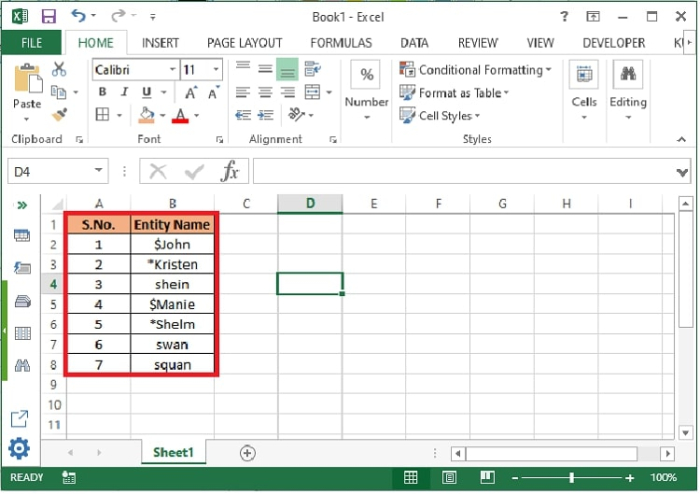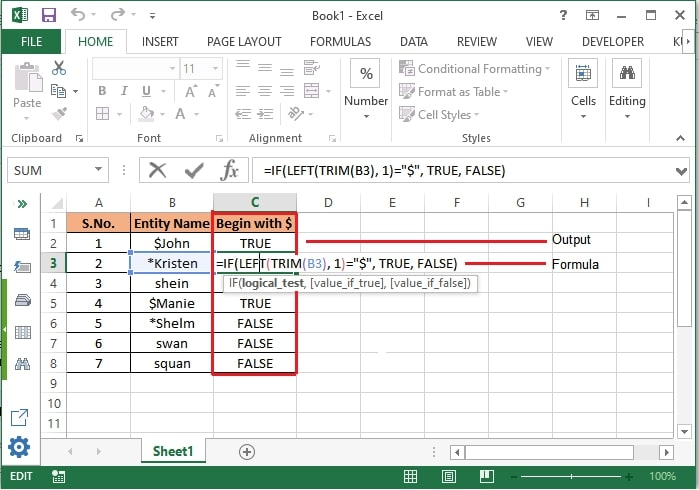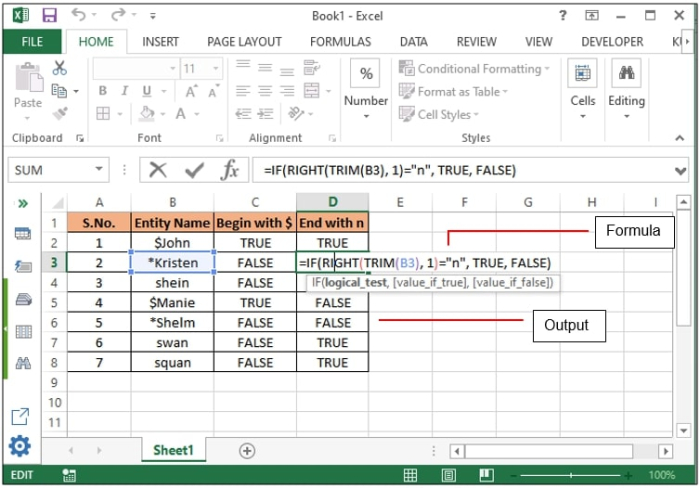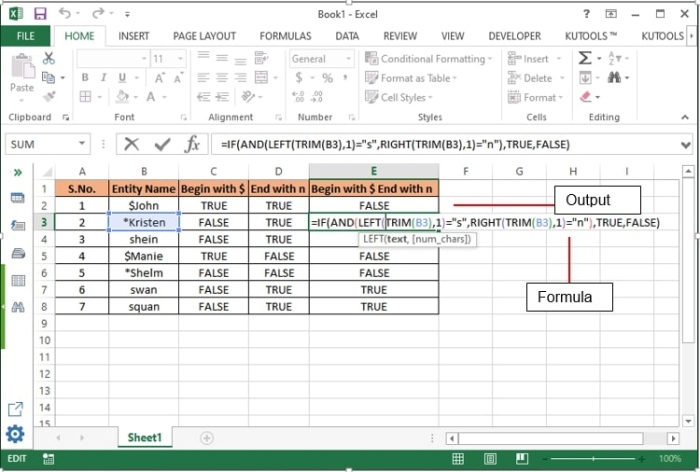# How To Check If Cell Begins Or Ends With A Specific Character In Excel?

When we have a list of entries in excel file and need to identify if there are some entities starting with a specific letter of special character, then looking at each value and counting will be a tedious task to do. This article will help you to find those entities using a formula without looking at each value of the list.

## Searching Cells containing Specific Character using Formula

### Searching the cells starting with a specific character

Let’s take an example of the following data −Now, if you want to check the cells begin with the character “$” then follow the below steps − Step 1 − Create a column where you want to the output to be displayed against each cell. Step 2 − Enter the following formula by selecting the required cell whose value to be scanned and press enter. =IF(LEFT(TRIM(B2),1)="$","True”, “False")Step 3 − Now drag the cell to copy the formula in other cells also to view the output against all cells.

Formula Syntax Description

Argument Description
IF(logical_test, {value_if_true},{value_if_false}
• Logical_test specifies the condition basis which the data needs to be rendered.

• Value_if_true specifies the value that shall be returned if the condition satisfies.

• Value_if_false specifies the value that shall be returned if the condition does not satisfy.

LEFT(text, [num_chars])
• Text specifies the cell address whose value to be returned or scanned from left.

• num_chars specifies the number of characters from left that need to be returned/scanned.

TRIM(
• Removes all extra spaces from the string of selected cell except for single spaces between words.

### Searching the cells ending with a specific character

Now, if you want to check the cells ends with the character “n” then follow the below steps −

Step 1 − Create a column where you want to the output to be displayed against each cell.

Step 2 − Enter the following formula by selecting the required cell whose value to be scanned and press enter.

=IF(RIGHT(TRIM(B2),1)="n","True”, “False")Step 3 − Now drag the cell to copy the formula in other cells also to view the output against all cells.

Formula Syntax Description

Argument Description
RIGHT(text, [num_chars])
• Text specifies the cell address whose value to be returned or scanned from right

• num_chars specifies the number of characters from left that need to be returned/scanned.

### Searching the cells starting and ending with a specific character

Now, if you want to check the cells starting and ending with the character “s” and “n” respectively,then follow the below steps −

Step 1 − Create a column where you want to the output to be displayed against each cell.

Step 2 − Enter the following formula by selecting the required cell whose value to be scanned and press enter.

=IF(AND(LEFT(TRIM(B3),1)="s",RIGHT(TRIM(B3),1)="n"),TRUE,FALSE)Step 3 − Now drag the cell to copy the formula in other cells also to view the output against all cells.

Formula Syntax Description

Argument Description
AND
• This operator is used in IF statement when two conditions simultaneously need to be verified. Similarly OR (either) and NOT (not satisfied) conditions can be used.

## Conclusion

Hence, using the above-mentioned formulas any number of data can be verified for specific values. These formulas will reduce the processing time of a dataset and enhance the user experience and data visualization. Keep learning, keep exploring Excel.

Updated on: 16-Sep-2022

8K+ Views